Theory and Modern Applications

# Global Mittag-Leffler synchronization of delayed fractional-order memristive neural networks

## Abstract

This paper deals with the global Mittag-Leffler synchronization of fractional-order memristive neural networks (FMNNs) with time delay. Since the FMNNs are essentially a class of switched systems with irregular switching laws, it is more difficult to achieve synchronization than with the traditional neural networks. First, under the framework of fractional-order differential inclusions and set-valued maps, the FMNNs are transformed into a continuous system with uncertainties. Then a linear state feedback combined with switching control law is designed in order to achieve the Mittag-Leffler synchronization. In addition, several synchronization criteria are obtained by constructing appropriate Lyapunov functionals, together with the help of some inequality techniques. Finally, an example is given to demonstrate the effectiveness of the obtained results.

## 1 Introduction

The memristor was first postulated by Chua in 1971, as the fourth fundamental circuit element together with the resistor, inductor and capacitor . In 2008, the Williams group announced a successful fabrication of a very compact and nonvolatile nano scale memory, called memristor , which describes the relationship between the magnetic flux and charge. If supplying current and voltage to the memristor, the resistance value of the memristor, (i.e., memristance) can be changed. When the voltage is turned off, the memristor remembers its most recent resistance value until the next time it is turned on. As shown in Fig. 1, it has been found that memristors exhibit the feature of pinched hysteresis, which is very similar to the behavior of neurons in the human brain. Owing to this special characteristic, memristor can be used in artificial neural networks to imitate the synapses. It inspires more and more researchers to replace resistors in traditional neural networks (NNs) by memristors to implement synaptic connection weights between neurons . Thus, the so-called memristive neural networks (MNNs) have been established. Since then considerable efforts have been made devoted to the investigation of MNNs, such as stability , stabilization , nonlinear dynamics analysis , and synchronization .

On the other hand, fractional-order dynamical systems have attracted considerable research interests due to their widespread applications in many fields such as neural systems  and viscoelastic systems . Compared with integer-order systems, a distinguishing feature of fractional-order systems is that they have long-term memory effects, hence it is suitable for describing various materials and processing more accurately . In the field of electronics, the model of a fractional capacitor has been presented . A fractional capacitor describes the fractional differentiation constitutive relationshipbetween the voltage $$V(t)$$ and the current $$I(t)$$ passing through it [23, 24]. In the past few years, many researchers have incorporated fractional capacitors into conventional NNs to establish the fractional-order neural networks (FNNs) model and to improve the accuracy of NN models. In consequence, some excellent results on the dynamical analysis of FNNs have been developed in .

In recent years, FMNNs have been proposed by replacing the common resistors in circuit implementation of FNNs by memristors to emulate synapses in the human brain. It is evident that FMNN models provide a significant improvement on FNNs. Therefore, the research on FMNNs has become a topic of focus and attracted increasing attention of many researchers. However, many traditional analysis methods, which are applicable to integer-order chaotic systems, cannot be extended and applied directly to FMNNs for the reason that FMNNs are represented by fractional-order differential equations (FDEs). In recent years, more and more scholars devoted their efforts to the dynamical analysis of FMNNs.

At present, some remarkable results relating to stability and synchronization of FMNNs have been reported in . For example, global Mittag-Leffler stability and synchronization have been investigated for FMNNs without time delay in . Projective synchronization of FMNNs has been discussed by constructing appropriate Lyapunov functions in . Adaptive synchronization of delayed FMNNs has been studied via delayed state feedback control in . Finite-time synchronization has been addressed for fractional-order memristive BAM neural networks via linear feedback control in . The synchronization issue for delayed FMNNs with parameter uncertainties or parameter mismatches has been discussed in  based on constructing suitable Lyapunov functionals together with the assistance of some fractional-order differential inequalities. Furthermore, synchronization of FMNNs with multiple delays has been discussed by using the maximum modulus principle . To the best of our knowledge, there are few results on global Mittag-Leffler synchronization of FMNNs with time delay.

Motivated by the aforementioned discussions, the main objective of this paper is to discuss the global Mittag-Leffler synchronization of delayed FMNNs. The main contributions of this paper are highlighted as follows. First, considering that FMNNs are described by FDEs with discontinuous right-hand sides, under the framework of fractional-order differential inclusions and set-valued maps, the FMNNs can be transformed into a continuous system with uncertainties. Second, a linear feedback combined with switching control law is designed, which plays an important role in the achievement of Mittag-Leffler synchronization. Third, several global Mittag-Leffler synchronization criteria are obtained for FMNNs by constructing suitable Lyapunov functionals. The obtained results extend and improve some previously published work on synchronization of FMNNs.

The rest of this paper is organized as follows. In Sect. 2, some lemmas, definitions and assumptions are introduced and the synchronization control schemes for FMNNs are designed. In Sect. 3, several global Mittag-Leffler synchronization criteria are derived. In Sect. 4, a numerical example is provided to illustrate the feasibility of the proposed theoretical results. Finally, some conclusions are drawn in Sect. 5.

## 2 Preliminaries and problem formulation

### Notations

Throughout this paper, the notation $$\|\cdot\|$$ is used to denote the 1-norm for vectors or for matrices, whenever appropriate. Besides, solutions of all the systems are considered in Filippov’s sense. C is the space of complex number, R is the space of real number, and $$R^{n}$$ denotes the n-dimensional Euclidean space. $$\operatorname{sign}(\cdot)$$ is the sign function. Let $$\tau> 0$$, $$C([-\tau, 0], R^{n})$$ denotes the family of continuous functions from $$[-\tau, 0]$$ to $$R^{n}$$.

### Definition 1

()

The Caputo fractional derivative of order α for a function $$f(t)$$ is defined aswhere $$t\geq t_{0}$$, and n is the positive integer such that $$n-1<\alpha<n$$. In particular, when $$0<\alpha<1$$, one has### Definition 2

()

The fractional integral of order α for a function $$f(t)$$ is defined aswhere $$t\geq{t_{0}}$$, $$\alpha>0$$, and $$\Gamma(\cdot)$$ is the Gamma function, that is, $$\Gamma(\alpha)=\int_{0}^{+\infty} e^{-t}t^{\alpha-1} \,dt$$.

### Definition 3

()

The Mittag-Leffler function with one parameter is defined as

$$E_{\alpha}(z)=\sum_{k=0}^{\infty}\frac{z^{k}}{\Gamma(k\alpha+1)},$$

where $$\alpha>0$$, and $$z\in C$$.

The Mittag-Leffler function with two parameters is defined as

$$E_{\alpha,\beta}(z)=\sum_{k=0}^{\infty}\frac{z^{k}}{\Gamma(k \alpha +\beta)},$$

where $$\alpha>0$$, $$\beta>0$$. When $$\beta=1$$, one has $$E_{\alpha ,1}(z)=E_{\alpha}(z)$$, and when $$\alpha=1$$, $$\beta=1$$, one further has $$E_{1,1}(z)=e^{z}$$.

### Lemma 1

()

For $$\alpha\in(0,1)$$, $$t \in(-\infty, +\infty)$$, we have

$$E_{\alpha}(t)>0,\qquad E_{\alpha, \alpha}(t)>0.$$

### Definition 4

()

The Laplace transform of Mittag-Leffler function with two parameters is

$$\mathcal{L} \bigl\{ t^{\beta-1}E_{\alpha,\beta}\bigl(-\lambda t^{\alpha}\bigr) \bigr\} =\frac{s^{\alpha-\beta}}{s^{\alpha}+\lambda},\quad \operatorname{Re}(s)> \vert \lambda \vert ^{1/ \alpha},$$

where t and s denote the variables in the time domain and Laplace domain, respectively; $$\operatorname{Re}(s)$$ denotes the real part of s, $$\lambda \in R$$, and $$\mathcal{L}$$ stands for the Laplace transform.

### Definition 5

()

Suppose $$E, Y\subset R^{n}$$, then $$F: E\rightarrow Y$$ is called a set-valued map, if for each point $$x\in E$$, there corresponds a nonempty set $$F(x)\subset Y$$. A set-valued map F with nonempty values is said to be upper-semi-continuous at $$x_{0} \in E \subset R^{n}$$ if, for any open set N containing $$F(x_{0})$$, there exists a neighborhood M of $$x_{0}$$ such that $$F(M)\subset N$$. $$F(x)$$ is said to have a closed (convex, compact) image if, for each $$x \in E$$, F(x) is closed (convex, compact).

### Definition 6

()

Consider the system $$\frac{dx}{dt}=g(x)$$, $$x \in R^{n}$$, with discontinuous right-hand sides, a set-valued map is defined as

$$\phi(x)=\bigcap_{\delta>0} \bigcap _{\mu(N)=0} K\bigl[g\bigl(B(x, \delta )\setminus N\bigr)\bigr],$$

where $$K[E]$$ is the closure of the convex hull of set E, $$B(x, \delta )=\{y: \|y-x\| \leq\delta\}$$, and $$\mu(N)$$ is the Lebesgue measure of the set N. A solution in Filippov’s sense of the Cauchy problem for this system with initial condition $$x(0)=x_{0}$$ is an absolutely continuous function $$x(t)$$, $$t \in[0, T]$$, which satisfies $$x(0)=x_{0}$$ and differential inclusion:

$$\frac{dx}{dt}\in\phi(x)$$

for a.e. $$t \in[0, T]$$.

### Lemma 2

()

If $$x(t) \in C^{1}([0, + \infty), R)$$ denotes a continuously differentiable function, for any $$\alpha\in(0, 1)$$, the following inequality holds almost everywhere:### 2.2 Problem formulation

In this paper, we consider a class of delayed FMNNs as drive system, which is described by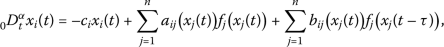(1)

where $$i=1, 2, \ldots, n$$, n is the number of neurons; $$c_{i}>0$$ denotes the self-feedback connection weight; $$0<\alpha<1$$ is the fractional order; $$x_{i}(t)$$ corresponds to the state variable connected with the ith neuron; τ is the time delay; $$f_{j}(\cdot)$$ denotes the activation function satisfying $$f_{j}(0)=0$$; $$a_{ij}(x_{j}(t))$$ and $$b_{ij}(x_{j}(t))$$ are memristive synaptic connection weights, which are defined by

$$a_{ij}\bigl(x_{j}(t)\bigr)=\frac{W_{fij}}{C_{i}}\times \delta_{ij},\qquad b_{ij}\bigl(x_{j}(t)\bigr)= \frac{W_{gij}}{C_{i}}\times\delta_{ij},$$

in which $$\delta_{ij}=1$$, if $$i\neq j$$ holds, otherwise, $$\delta _{ij}=-1$$. $$W_{fij}$$ and $$W_{gij}$$ denote the memductance of memristors $$R_{fij}$$ and $$R_{gij}$$, respectively. In , it has been shown that, since digital computer applications require only two memory states, memristor needs to display only two sufficiently different equilibrium states. Hence, the memristive synaptic connection weights, in view of the pinched hysteresis curve, can be simply represented as

$$a_{ij}\bigl(x_{j}(t)\bigr)= \textstyle\begin{cases} a_{ij}^{*},& |x_{j}(t)|\leq T_{j}, \\ a_{ij}^{**},& |x_{j}(t)|> T_{j} , \end{cases}\displaystyle \qquad b_{ij}\bigl(x_{j}(t) \bigr)= \textstyle\begin{cases} b_{ij}^{*},& |x_{j}(t)|\leq T_{j},\\ b_{ij}^{**},& |x_{j}(t)|> T_{j}, \end{cases}$$

for $$i, j=1, 2, \ldots, n$$, where the switching jump $$T_{j}>0$$, and $$a_{ij}^{*}$$, $$a_{ij}^{**}$$, $$b_{ij}^{*}$$, $$b_{ij}^{**}$$ are all constants. The initial condition of system (1) is assumed to be $$x_{i}(s)=\vartheta_{xi}(s)$$, $$s \in[-\tau, 0]$$, where $$\vartheta _{xi}(s) \in C([-\tau, 0], R)$$.

The response system is described by(2)

where $$i=1, 2, \ldots, n$$, $$c_{i}>0$$ denotes the self-feedback connection weight; $$y_{i}(t)$$ corresponds to the state variable connected with the ith neuron; $$u_{i}(t)$$ is the control input to be designed later.

Note that system (1) or (2) can be regarded as a class of discontinuous fractional-order differential equations because the memristive synaptic connection weights $$a_{ij}(x_{j}(t))$$, $$b_{ij}(x_{j}(t))$$, $$a_{ij}(y_{j}(t))$$, $$b_{ij}(y_{j}(t))$$ are all discontinuous. Hence, system (1) or (2) cannot be solved within the framework of classical Cauchy initial problem because the classical solutions to ordinary differential equations are not available. In this case, the solutions should be considered in Filippov’s sense , which is a useful tool to deal with differential equations with discontinuous right-hand sides. Based on the theory of set-valued maps and differential inclusions, from (1) and (2), we have(3)

and(4)

where

\begin{aligned}& K\bigl[a_{ij}\bigl(x_{j}(t)\bigr)\bigr]= \textstyle\begin{cases} a_{ij}^{*},& |x_{j}(t)|< T_{j},\\ \operatorname{co}\{a_{ij}^{*}, a_{ij}^{**}\},& |x_{j}(t)|= T_{j},\\ a_{ij}^{**},& |x_{j}(t)|> T_{j}, \end{cases}\displaystyle \\ & K\bigl[b_{ij}\bigl(x_{j}(t)\bigr)\bigr]= \textstyle\begin{cases} b_{ij}^{*},& |x_{j}(t)|< T_{j},\\ \operatorname{co}\{b_{ij}^{*}, b_{ij}^{**}\},& |x_{j}(t)|= T_{j},\\ b_{ij}^{**},& |x_{j}(t)|> T_{j}, \end{cases}\displaystyle \\ & K\bigl[a_{ij}\bigl(y_{j}(t)\bigr)\bigr]= \textstyle\begin{cases} a_{ij}^{*},& |y_{j}(t)|< T_{j},\\ \operatorname{co}\{a_{ij}^{*}, a_{ij}^{**}\},& |y_{j}(t)|= T_{j},\\ a_{ij}^{**},& |y_{j}(t)|> T_{j} , \end{cases}\displaystyle \\ & K\bigl[b_{ij}\bigl(y_{j}(t)\bigr)\bigr]= \textstyle\begin{cases} b_{ij}^{*},& |y_{j}(t)|< T_{j},\\ \operatorname{co}\{b_{ij}^{*}, b_{ij}^{**}\},& |y_{j}(t)|= T_{j},\\ b_{ij}^{**},& |y_{j}(t)|> T_{j}, \end{cases}\displaystyle \end{aligned}

for $$i, j=1, 2, \ldots, n$$, and $$\operatorname{co}\{a_{ij}^{*}, a_{ij}^{**}\} =[\underline{a}_{ij}, \overline{a}_{ij}]$$, $$\operatorname{co}\{ b_{ij}^{*}, b_{ij}^{**}\}=[\underline{b}_{ij}, \overline{b}_{ij}]$$, $$\overline{a}_{ij}=\max\{a_{ij}^{*}, a_{ij}^{**} \}$$, $$\underline{a}_{ij}=\min\{ a_{ij}^{*}, a_{ij}^{**}\}$$, $$\overline{b}_{ij}=\max\{b_{ij}^{*}, b_{ij}^{**} \}$$, $$\underline{b}_{ij}=\min\{ b_{ij}^{*}, b_{ij}^{**}\}$$.

Based on the measurable selection theorem in , there exist measurable functions $$\gamma_{ij}(x_{j}(t))\in K[a_{ij}(x_{j}(t))]$$, $$\chi_{ij}(x_{j}(t))\in K[b_{ij}(x_{j}(t))]$$, $$\gamma _{ij}(y_{j}(t))\in K[a_{ij}(y_{j}(t))]$$, $$\chi_{ij}(y_{j}(t))\in K[b_{ij}(y_{j}(t))]$$ such that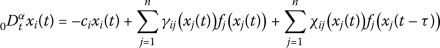(5)

and(6)

To ensure the existence of the solutions of systems (5) and (6), respectively, throughout this paper, we assume that the activation function $$f_{j}(\cdot)$$ satisfies the following properties.

### Assumption 1

The activation function $$f_{j}(\cdot)$$ is Lipschitz-continuous with Lipschitz constant $$L_{j}> 0$$, i.e.,

$$\bigl\vert f_{j}(u)-f_{j}(v) \bigr\vert \leq L_{j} |u-v|,$$

for all $$u ,v \in R$$, and $$j=1,2,\ldots,n$$.

### Assumption 2

The activation function $$f_{j}(\cdot)$$ is bounded on R, i.e., there exists a constant $$M_{j}$$ such that

$$\bigl\vert f_{j}(u) \bigr\vert \leq M_{j}, \quad u \in R, j=1,2,\ldots,n .$$

Let $$e_{i}(t)=y_{i}(t)- x_{i}(t)$$ be the synchronization error and the controller can be designed as

$$u_{i}(t)=-k_{i} e_{i}(t) -\operatorname{sign} \bigl(e_{i}(t)\bigr)\epsilon_{i} \bigl\vert e_{i}(t-\tau) \bigr\vert - \rho_{i} \operatorname{sign} \bigl(e_{i}(t)\bigr).$$
(7)

where $$k_{i}$$, $$\epsilon_{i}$$, $$\rho_{i}$$ denote the feedback gains.

Then the error system can be given by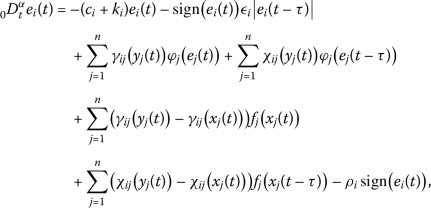(8)

where $$\varphi_{j}(e_{j}(t))=f_{j}(y_{j}(t))-f_{j}(x_{j}(t))$$.

The initial condition of error system (8) is defined by

$$e_{i}(s)=\phi_{i}(s), \quad -\tau\leq s\leq0,$$

where $$\phi_{i}(s)=\vartheta_{yi}(s)-\vartheta_{xi}(s)\in C([-\tau ,0],R)$$, $$\phi(s)=[\phi_{1}(s), \phi_{2}(s), \ldots, \phi_{n}(s)]^{T}$$.

In the next section, global Mittag-Leffler synchronization of drive–response systems (5)–(6) will be achieved. The definition of global Mittag-Leffler stability is as follows.

### Definition 7

()

The zero solution of the error system (8) is said to be globally Mittag-Leffler stable if there exist two positive constants N and λ such that, for any solutions $$\phi(t)$$ of error system (8) with different initial condition denoted by $$\phi(0)$$, one has

$$\bigl\Vert \phi(t) \bigr\Vert \leq N \bigl\Vert \phi(0) \bigr\Vert E_{\alpha}\bigl(-\lambda t^{\alpha}\bigr),\quad t \geq0.$$

## 3 Main result

### Theorem 1

Suppose Assumptions 12 hold and that the following algebraic conditions:

\begin{aligned} (\mathrm{i}) &\quad \min_{1 \leq i \leq n} \Biggl\{ c_{i}+k_{i}- \sum_{j=1}^{n}a_{ji}^{u}L_{i} \Biggr\} > 0 , \\ (\mathrm{ii}) &\quad \varepsilon_{i}- \sum _{j=1}^{n}b_{ji}^{u}L_{i} \geq0, \quad i=1, 2, \ldots, n, \\ (\mathrm{iii}) &\quad \rho_{i}- \sum_{j=1}^{n} \bigl( \bigl\vert a_{ij}^{*}-a_{ij}^{**} \bigr\vert + \bigl\vert b_{ij}^{*}-b_{ij}^{**} \bigr\vert \bigr)M_{j} \geq0, \quad i=1, 2, \ldots, n, \end{aligned}

are satisfied, then error system (8) is globally Mittag-Leffler stable, where $$a_{ji}^{ u}=\max\{ |a_{ji}^{*}|, |a_{ji}^{**}|\}$$, $$b_{ji}^{u}=\max\{ |b_{ji}^{*}|, |b_{ji}^{**}|\}$$, $$i, j=1, 2, \ldots, n$$. That is, the response system (2) under the controller (7) can be globally Mittag-Leffler synchronized with the drive system (1).

### Proof

Construct the Lyapunov functional as follows:

$$V(t)=\sum_{i=1}^{n} \bigl\vert e_{i}(t) \bigr\vert .$$

From Lemma 2 and Assumptions 12, we obtain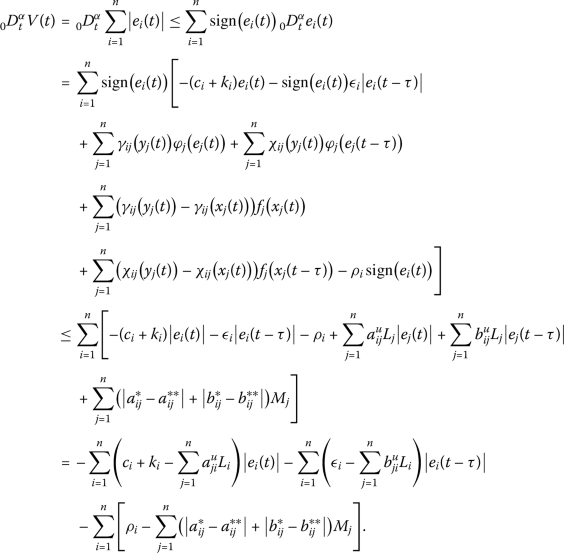Based on the conditions (i)–(iii) of Theorem 1, we have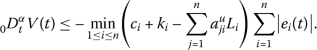Let $$\lambda=\min_{1 \leq i \leq n} \{ c_{i}+k_{i}-\sum_{j=1}^{n}a_{ji}^{ u} L_{i} \}$$, we haveThus, there exists a nonnegative function $$F(t)$$ satisfying(9)

Taking the Laplace transform on both sides of (9), it follows that

$$V(s)=\frac{s^{\alpha-1}}{s^{\alpha}+\lambda}V(0)- \frac {F(s)}{s^{\alpha}+\lambda},$$

where $$V(s)= \mathcal{L}\{ V(t) \}$$, $$F(s)= \mathcal{L}\{ F(t) \}$$.

By the inverse Laplace transform, we obtain

$$V(t) = V(0)E_{\alpha}\bigl(-\lambda t^{\alpha}\bigr)- F(t)*t^{\alpha-1} E_{\alpha, \alpha}\bigl(-\lambda t^{\alpha}\bigr), \quad t\geq0,$$

where denotes the convolution operator. From Lemma 1, $$F(t)$$, $$t^{\alpha-1} E_{\alpha, \alpha}(-\lambda t^{\alpha})$$ are all nonnegative functions. Then we can obtain

$$V(t) \leq V(0)E_{\alpha}\bigl(-\lambda t^{\alpha}\bigr), \quad t\geq0.$$

That is,

$$\bigl\Vert e(t) \bigr\Vert \leq \bigl\Vert e(0) \bigr\Vert E_{\alpha}\bigl(-\lambda t^{\alpha}\bigr), \quad t \geq0.$$

By Definition 7, the error system (8) is globally Mittag-Leffler stable, which implies the response system (2) under the controller (7) can be globally Mittag-Leffler synchronized with the drive system (1). This completes the proof. □

If the drive–response systems are without time delay, then the drive–response systems (5)–(6) are reduced to the following two systems:(10)(11)

Accordingly, the controller is reduced to

$$u_{i}(t)=-k_{i} e_{i}(t) - \rho_{i} \operatorname{sign}\bigl(e_{i}(t)\bigr).$$
(12)

Then the error system can be given by(13)

where $$\varphi_{j}(e_{j}(t))=f_{j}(y_{j}(t))-f_{j}(x_{j}(t))$$.

### Corollary 1

Suppose Assumptions 12 hold and that the following algebraic conditions:

\begin{aligned} (\mathrm{i})&\quad \min_{1 \leq i \leq n} \Biggl\{ c_{i}+k_{i}- \sum_{j=1}^{n}a_{ji}^{u}L_{i} \Biggr\} > 0 , \\ (\mathrm{ii})&\quad \rho_{i}- \sum_{j=1}^{n} \bigl( \bigl\vert a_{ij}^{*}-a_{ij}^{**} \bigr\vert \bigr)M_{j} \geq0 , \quad i=1, 2, \ldots, n, \end{aligned}

are satisfied, then error system (13) is globally Mittag-Leffler stable, where $$i, j=1, 2, \ldots, n$$, $$a_{ji}^{ u}=\max \{ |a_{ji}^{*}|, |a_{ji}^{**}|\}$$. That is, the response system (11) under the controller (12) can be globally Mittag-Leffler synchronized with the drive system (10).

### Remark 1

In controller (7), the terms $$- \rho_{i} \operatorname{sign}(e_{i}(t))$$ play an important role in eliminating the undesired error caused by the memristive synaptic connection weights. In order to achieve the global Mittag-Leffler synchronization, the feedback gains $$\rho_{i}$$ should be well designed such that $$\rho_{i}- \sum_{j=1}^{n} (|a_{ij}^{*}-a_{ij}^{**}| + |b_{ij}^{*}-b_{ij}^{**}| )M_{j} \geq0$$ are ensured. It also implies that, besides the Lipschitz-continuous condition, boundedness constraint should be imposed on the activation functions. In our future work, we will discuss how to reduce the conservatism of the activation functions.

### Remark 2

In this paper, by constructing appropriate Lyapunov functionals and designing suitable hybrid controllers, some sufficient criteria are established to achieve the globally Mittag-Leffler synchronization of delayed FMNNs. Notice that the memristive synaptic connection weights $$a_{ij}(\cdot)$$, $$b_{ij}(\cdot)$$ are both state-dependent, which makes it impossible to achieve the complete synchronization only via linear state feedback control. To this end, $$-\operatorname{sign}(e_{i}(t))\epsilon _{i}|e_{i}(t-\tau)|$$, and $$-\rho_{i} \operatorname{sign}(e_{i}(t))$$ are added to the linear state feedback to deal with the delay term and the undesirable error caused by the differences between the memristive synaptic connection weights. Actually, there are few results relevant to the global Mittag-Leffler synchronization of delayed FMNNs (1) in the current literature. Therefore, the obtained results in this paper can fill this gap.

### Remark 3

FMNNs are a class of switched systems with $$2^{n}$$ FNN subsystems. It should be mentioned that the memristive synaptic connection weights of FMNNs, i.e., $$a_{ij}(x_{j}(t))$$, $$a_{ij}(y_{j}(t))$$, $$b_{ij}(x_{j}(t))$$, and $$b_{ij}(y_{j}(t))$$ are time-varying; they which are dependent on the corresponding system states $$x_{j}(t)$$ and $$y_{j}(t)$$. Hence, compared with the traditional FNNs , it is more difficult to realize the synchronization of FMNNs. In this paper, a linear state feedback combined with switching control law is designed to overcome this difficulty. It is believed that the obtained results are useful and effective for the designs and applications of FMNNs.

## 4 Numerical simulations

In this section, the Adams–Bashforth–Moulton predictor–corrector algorithm is applied in the numerical simulations [44, 45].

Consider the drive system (1) with $$n=3$$, $$\alpha=0.9$$, $$f_{j}(x_{j})=\tanh(x_{j})$$, $$\tau=0.8$$, initial condition $$\vartheta _{x}(s)=(0.3, -0.6, 0.2)^{T}$$, $$s \in[-0.8, 0]$$. Set $$c_{1}=2.2$$, $$c_{2}=1.2$$, $$c_{3}=1.8$$,

\begin{aligned}& a_{11}(x_{1})= \textstyle\begin{cases} 2.2,& |x_{1}|\leq1,\\ 2,& |x_{1}|> 1, \end{cases}\displaystyle \qquad a_{12}(x_{2})= \textstyle\begin{cases} -2,& |x_{2}|\leq1,\\ -2.1,& |x_{2}|> 1, \end{cases}\displaystyle \\& a_{13}(x_{3})= \textstyle\begin{cases} 2,& |x_{3}|\leq1,\\ 1.8,& |x_{3}|> 1, \end{cases}\displaystyle \qquad a_{21}(x_{1})= \textstyle\begin{cases} -0.8,& |x_{1}|\leq1,\\ -0.6,& |x_{1}|> 1, \end{cases}\displaystyle \\& a_{22}(x_{2})= \textstyle\begin{cases} 5.71,& |x_{2}|\leq1,\\ 5.68,& |x_{2}|> 1, \end{cases}\displaystyle \qquad a_{23}(x_{3})= \textstyle\begin{cases} 1.15,& |x_{3}|\leq1,\\ 1.1,& |x_{3}|> 1, \end{cases}\displaystyle \\& a_{31}(x_{1})= \textstyle\begin{cases} -4.75,& |x_{1}|\leq1,\\ -4.5,& |x_{1}|> 1, \end{cases}\displaystyle \qquad a_{32}(x_{2})= \textstyle\begin{cases} -1,& |x_{2}|\leq1,\\ -0.8,& |x_{2}|> 1, \end{cases}\displaystyle \\& a_{33}(x_{3})= \textstyle\begin{cases} 1.2,& |x_{3}|\leq1,\\ 1.25,& |x_{3}|> 1, \end{cases}\displaystyle \qquad b_{11}(x_{1})= \textstyle\begin{cases} -4,& |x_{1}|\leq1,\\ -3.8,& |x_{1}|> 1, \end{cases}\displaystyle \\& b_{12}(x_{2})= \textstyle\begin{cases} 2.5,& |x_{2}|\leq1,\\ 2.3,& |x_{2}|> 1, \end{cases}\displaystyle \qquad b_{13}(x_{3})= \textstyle\begin{cases} -3.2,& |x_{3}|\leq1,\\ -3.0,& |x_{3}|> 1, \end{cases}\displaystyle \\& b_{21}(x_{1})= \textstyle\begin{cases} -1.5,& |x_{1}|\leq1,\\ -1.7,& |x_{1}|> 1, \end{cases}\displaystyle \qquad b_{22}(x_{2})= \textstyle\begin{cases} -3.6,& |x_{2}|\leq1,\\ -3.8,& |x_{2}|> 1, \end{cases}\displaystyle \\& b_{23}(x_{3})= \textstyle\begin{cases} -2.3,& |x_{3}|\leq1,\\ -2.5,& |x_{3}|> 1, \end{cases}\displaystyle \qquad b_{31}(x_{1})= \textstyle\begin{cases} 0.3,& |x_{1}|\leq1,\\ 0.4,& |x_{1}|> 1, \end{cases}\displaystyle \\& b_{32}(x_{2})= \textstyle\begin{cases} 1.8,& |x_{2}|\leq1,\\ 2,& |x_{2}|> 1, \end{cases}\displaystyle \qquad b_{33}(x_{3})= \textstyle\begin{cases} 1.2,& |x_{3}|\leq1,\\ 1.5,& |x_{3}|> 1. \end{cases}\displaystyle \end{aligned}

The response system (2) is assumed to have the same parameters as those for the drive system, except for the different initial condition $$\vartheta_{y}(s)=(-2, -3, 2)^{T}$$, $$s \in[-0.8, 0]$$.

Figure 2(a) and Fig. 2(b) depict the phase portraits of the $$x_{2}$$$$x_{1}$$ plane and $$x_{2}$$$$x_{3}$$ plane of the drive system, respectively. When the controller (7) is at rest, that is, $$k_{1}=k_{2}=k_{3}=0$$, $$\epsilon_{1}=\epsilon_{2}=\epsilon_{3}=0$$, $$\rho_{1}=\rho _{2}=\rho_{3}=0$$, the switching laws of the memristive synaptic connection weights $$a_{11}(x_{1})$$, $$a_{11}(y_{1})$$ are shown in Fig. 3(a) and Fig. 3(b), respectively. From Fig. 3, one can see that the switching laws of $$a_{11}(x_{1})$$, $$a_{11}(y_{1})$$ evoked by the nonidentical initial conditions are different from each other.

Taking $$k_{1}=5.56$$, $$k_{2}=7.62$$, $$k_{3}=2.61$$, $$\epsilon_{1}=6.1$$, $$\epsilon _{2}=8.3$$, $$\epsilon_{3}=7.2$$, $$\rho_{1}=1.1$$, $$\rho_{2}=0.88$$, $$\rho_{3}=1.1$$, by simple calculation, we can obtain $$\lambda=\min_{1 \leq i \leq3} \{ c_{i}+k_{i}- \sum_{j=1}^{3}a_{ji}^{u}L_{i} \}=0.01 > 0$$, $$\varepsilon_{i}- \sum_{j=1}^{3}b_{ji}^{u}L_{i}=0$$, $$\rho_{i}- \sum_{j=1}^{3} (|a_{ij}^{*}-a_{ij}^{**}| + |b_{ij}^{*}-b_{ij}^{**}| )M_{j} =0$$. It means that the conditions of Theorem 1 are satisfied. According to Theorem 1, we can obtain the following inequality:

$$\bigl\Vert e(t) \bigr\Vert \leq \bigl\Vert e(0) \bigr\Vert E_{0.9} \bigl(-0.01t^{0.9}\bigr), \quad t\geq0.$$

Then the error system (8) is globally Mittag-Leffler stable, which is verified by Fig. 4. From Fig. 5, the switching laws of $$a_{11}(x_{1})$$, $$a_{11}(y_{1})$$, under the designed controller (7), become identical as time evolves. Choose six random initial values, Fig. 6 depicts the time evolution of synchronization error $$e_{1}(t)$$, $$e_{2}(t)$$, $$e_{3}(t)$$. From Figs. 46, one can find that the drive–response systems (1)–(2) can achieve globally Mittag-Leffler synchronization under controller (7). The numerical simulations verify the effectiveness of the proposed method.

## 5 Conclusions

In this paper, the global Mittag-Leffler synchronization of delayed FMNNs is investigated. A linear state feedback control combined with switching control law is designed, which is simple and easy to implement. Two Mittag-Leffler synchronization criteria are developed by constructing suitable Lyapunov functionals. It is the first time that the global Mittag-Leffler synchronization is discussed under the proposed controller. The effectiveness of the developed method has been demonstrated theoretically and numerically.

## References

1. Chua, L.: Memristor—the missing circuit element. IEEE Trans. Circuit Theory 18(5), 507–519 (1971)

2. Strukov, D.B., Snider, G.S., Stewart, D.R., Williams, R.S.: The missing memristor found. Nature 453(7191), 80–83 (2008)

3. Jo, S.H., Chang, T., Ebong, I., Bhadviya, B.B., Mazumder, P., Lu, W.: Nanoscale memristor device as synapse in neuromorphic systems. Nano Lett. 10(4), 1297–1301 (2010)

4. Sah, M.P., Yang, C., Kim, H., Chua, L.O.: A voltage mode memristor bridge synaptic circuit with memristor emulators. Sensors 12(3), 3587–3604 (2012)

5. Adhikari, S.P., Yang, C., Kim, H., Chua, L.O.: Memristor bridge synapse-based neural network and its learning. IEEE Trans. Neural Netw. Learn. Syst. 23(9), 1426–1435 (2012)

6. Itoh, M., Chua, L.O.: Memristor cellular automata and memristor discrete-time cellular neural networks. Int. J. Bifurc. Chaos 19(11), 3605–3656 (2009)

7. Bao, G., Zeng, Z.: Region stability analysis for switched discrete-time recurrent neural network with multiple equilibria. Neurocomputing 249, 182–190 (2017)

8. Zhang, G., Shen, Y.: Exponential stabilization of memristor-based chaotic neural networks with time-varying delays via intermittent control. IEEE Trans. Neural Netw. Learn. Syst. 26(7), 1431–1441 (2015)

9. Wu, A., Zeng, Z.: Global Mittag-Leffler stabilization of fractional-order memristive neural networks. IEEE Trans. Neural Netw. Learn. Syst. 28(1), 206–217 (2017)

10. Wang, L., Shen, Y., Zhang, G.: Finite-time stabilization and adaptive control of memristor-based delayed neural networks. IEEE Trans. Neural Netw. Learn. Syst. 28(11), 2648–2659 (2017)

11. Fan, Y., Huang, X., Wang, Z., Li, Y.: Nonlinear dynamics and chaos in a simplified memristor-based fractional-order neural network with discontinuous memductance function. Nonlinear Dyn. 93(2), 611–627 (2018)

12. Abdurahman, A., Jiang, H., Teng, Z.: Finite-time synchronization for memristor-based neural networks with time-varying delays. Neural Netw. 69, 20–28 (2015)

13. Li, N., Cao, J.: Lag synchronization of memristor-based coupled neural networks via ω-measure. IEEE Trans. Neural Netw. Learn. Syst. 27(3), 686–697 (2016)

14. Yang, X., Ho, D.W.: Synchronization of delayed memristive neural networks: robust analysis approach. IEEE Trans. Cybern. 46(12), 3377–3387 (2016)

15. Xin, Y., Li, Y., Huang, X., Cheng, Z.: Quasi-synchronization of delayed chaotic memristive neural networks. IEEE Trans. Cybern. (2017). https://doi.org/10.1109/TCYB.2017.2765343

16. Yang, X., Cao, J., Liang, J.: Exponential synchronization of memristive neural networks with delays: interval matrix method. IEEE Trans. Neural Netw. Learn. Syst. 28(8), 1878–1888 (2017)

17. Yang, X., Li, C., Huang, T., Song, Q., Huang, J.: Synchronization of fractional-order memristor-based complex-valued neural networks with uncertain parameters and time delays. Chaos Solitons Fractals 110, 105–123 (2018)

18. Fan, Y., Huang, X., Li, Y., Xia, J., Chen, G.: Aperiodically intermittent control for quasi-synchronization of delayed memristive neural networks: an interval matrix and matrix measure combined method. IEEE Trans. Syst. Man Cybern. Syst. (2018). https://doi.org/10.1109/TSMC.2018.2850157

19. Lundstrom, B.N., Higgs, M.H., Spain, W.J., Fairhall, A.L.: Fractional differentiation by neocortical pyramidal neurons. Nat. Neurosci. 11(11), 1335–1342 (2008)

20. Tripathi, D., Pandey, S.K., Das, S.: Peristaltic flow of viscoelastic fluid with fractional Maxwell model through a channel. Appl. Math. Comput. 215(10), 3645–3654 (2010)

21. Samko, S.G., Kilbas, A.A., Marichev, O.I.: Fractional Integrals and Derivatives and Some of Their Applications. Nauka i Technika, Minsk (1987)

22. Nakagawa, M., Sorimachi, K.: Basic characteristics of a fractance device. IEICE Trans. Fundam. Electron. Commun. Comput. Sci. 75(12), 1814–1819 (1992)

23. Westerlund, S., Ekstam, L.: Capacitor theory. IEEE Trans. Dielectr. Electr. Insul. 1(5), 826–839 (1994)

24. Boroomand, A., Menhaj, M.: Fractional-order Hopfield neural networks. In: Advances in Neuro-Information Processing. Lecture Notes in Computer Science, vol. 5506, pp. 883–890. Springer, Berlin (2009)

25. Zhang, S., Yu, Y., Yu, J.: LMI conditions for global stability of fractional-order neural networks. IEEE Trans. Neural Netw. Learn. Syst. 28(10), 2423–2433 (2017)

26. Wang, Z., Wang, X., Li, Y., Huang, X.: Stability and Hopf bifurcation of fractional-order complex-valued single neuron model with time delay. Int. J. Bifurc. Chaos 27(13), 1750209 (2017)

27. Wang, H., Yu, Y., Wen, G., Zhang, S., Yu, J.: Global stability analysis of fractional-order Hopfield neural networks with time delay. Neurocomputing 154, 15–23 (2015)

28. Ding, Z., Shen, Y.: Projective synchronization of nonidentical fractional-order neural networks based on sliding mode controller. Neural Netw. 76, 97–105 (2016)

29. Chen, J., Zeng, Z., Jiang, P.: Global Mittag-Leffler stability and synchronization of memristor-based fractional-order neural networks. Neural Netw. 51, 1–8 (2014)

30. Bao, H.B., Cao, J.D.: Projective synchronization of fractional-order memristor-based neural networks. Neural Netw. 63, 1–9 (2015)

31. Bao, H., Park, J.H., Cao, J.: Adaptive synchronization of fractional-order memristor-based neural networks with time delay. Nonlinear Dyn. 82(3), 1343–1354 (2015)

32. Xiao, J., Zhong, S., Li, Y., Xu, F.: Finite-time Mittag-Leffler synchronization of fractional-order memristive BAM neural networks with time delays. Neurocomputing 219, 431–439 (2017)

33. Gu, Y., Yu, Y., Wang, H.: Synchronization for fractional-order time-delayed memristor-based neural networks with parameter uncertainty. J. Franklin Inst. 353(15), 3657–3684 (2016)

34. Huang, X., Fan, Y., Jia, J., Wang, Z., Li, Y.: Quasi-synchronisation of fractional-order memristor-based neural networks with parameter mismatches. IET Control Theory Appl. 11(14), 2317–2327 (2017)

35. Fan, Y., Huang, X., Wang, Z., Li, Y.: Global dissipativity and quasi-synchronization of asynchronous updating fractional-order memristor-based neural networks via interval matrix method. J. Franklin Inst. 355(13), 5998–6025 (2018)

36. Chen, L., Cao, J., Wu, R., Machado, J.T., Lopes, A.M., Yang, H.: Stability and synchronization of fractional-order memristive neural networks with multiple delays. Neural Netw. 94, 76–85 (2017)

37. Podlubny, I.: Fractional Differential Equations Academic Press, London (1999)

38. Wei, Z., Li, Q., Che, J.: Initial value problems for fractional differential equations involving Riemann–Liouville sequential fractional derivative. J. Math. Anal. Appl. 367(1), 260–272 (2010)

39. Henderson, J., Ouahab, A.: Fractional functional differential inclusions with finite delay. Nonlinear Anal. 70(5), 2091–2105 (2009)

40. Filippov, A.F.: Differential Equations with Discontinuous Righthand Sides. Kluwer Academic, Boston (1988)

41. Zhang, S., Yu, Y., Wang, H.: Mittag-Leffler stability of fractional-order Hopfield neural networks. Nonlinear Anal. Hybrid Syst. 16, 104–121 (2015)

42. Chua, L.O.: Resistance switching memories are memristors. Appl. Phys. A 102(4), 765–783 (2011)

43. Aubin, J.P., Cellina, A.: Differential Inclusions: Set-Valued Maps and Viability Theory. Springer, Berlin (1984)

44. Wang, Z.: A numerical method for delayed fractional-order differential equations. J. Appl. Math. 2013, Article ID 256071 (2013)

45. Wang, Z., Huang, X., Zhou, J.: A numerical method for delayed fractional-order differential equations: based on G-L definition. Appl. Math. Inf. Sci. 7, 525–529 (2013)

### Acknowledgements

The authors would like to thank the referees for their valuable comments and suggestions.

Not applicable.

## Funding

This work was partially supported by the National Nature Science Foundation of China (Grant Nos. 61573008, 61473178 and 61473177), China Scholarship Council (Grant No. 201708210053), Post-Doctoral Applied Research Projects of Qingdao (No. 2016115) and SDUST Research Fund (No. 2014TDJH102).

## Author information

Authors

### Contributions

The authors declare that the study was realized in collaboration with the same responsibility. All authors read and approved the final manuscript.

### Corresponding author

Correspondence to Zhen Wang.

## Ethics declarations

### Competing interests

The authors declare that they have no competing interests.